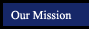Properties, Postulates and Theorems
textbook tutorials - definitions - constructions - postulates and theorems - internet activities and resources
 mathematical logic symmetry and similarity points, lines and planes parallel lines triangles quadrilaterals polygons circles 3-Dimensional Geometry
 Reflexive Property of Equality/Congruency Addition Property of = Symmetric Property of Equality/Congruency Subtraction Property of = Transitive Property of Equality/Congruency Multiplication Property of = Division Property of =